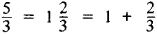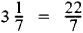# improper fraction

Also found in: Dictionary, Thesaurus, Legal, Wikipedia.

## improper fraction

a fraction in which the numerator has a greater absolute value or degree than the denominator, as 7/6 or (x2 + 3)/(x + 1)

## Improper Fraction

an arithmetic fraction whose numerator is greater than or equal to the denominator—for example, 5/3, 4/2, and 7/7. An improper fraction can be represented, by separating its integral part, in the form of a mixed number—that is, a number having an integral part and a fractional part, for example,Conversely, every mixed number can be written in the form of an improper fraction, for example,## improper fraction

[im′präp·ər ′frak·shən]
(mathematics)
In arithmetic, the quotient of two integers in which the numerator is greater than or equal to the denominator.
In algebra, the quotient of two polynomials in which the degree of the numerator is greater than or equal to that of the denominator.
Mentioned in ?
References in periodicals archive ?
Step 4: The result 4 is an improper fraction and must be reduced to a mixed number, which would be 1 1/4.
6, an equation 1 25 = 13/5 can be recognized as an error step of converting a mixed number to an improper fraction.
Students, who have only learned the shortcut algorithm for renaming mixed numbers as improper fractions, may not know that a mixed number is a sum.
The data from 323 interviews with students at the end of Year 6 (Clarke, Roche, Mitchell & Sukenik, 2006) indicated that students need classroom experiences which assist them to understand more clearly the roles of the numerator and denominator in a fraction, the meaning of improper fractions, and the relative sizes of fractions.
For example, an environment for solving linear equations can accept both decimal and common fractions or mixed numbers and improper fractions as answers.
My guys are close, but I need to go back to work on denominators with improper fractions.
These operations suggest that Joe was developing a scheme for generating improper fractions.
This came about because the author previously reviewed that the denominator meant "the number of pieces it takes to make a whole", rather than "the number of pieces in all" to clarify confusion and allow the definition to include improper fractions.
Varying the numbers in these problems produces a variety of fractions as solutions, including unit fractions, such as 1/3 for three children sharing one candy bar; nonunit fractions, such as 3/4 in the candy-bar problem; improper fractions, such as 10/4 for four children sharing ten candy bars; and mixed numbers, such as 2 1/6 for six children sharing thirteen candy bars.
As part of ACMNA078, students should become competent in "converting mixed numbers to improper fractions and vice versa" (ACARA, 2013, p.
She then counted the number of half units and labelled the tick marks using improper fractions on the number line.
This is a useful addition to the simplistic 'out of' feature since it helps us make sense of improper fractions like thirteen-tenths.

Site: Follow: Share:
Open / Close